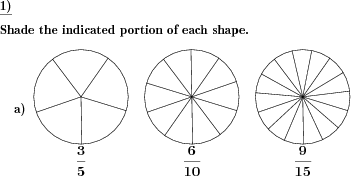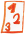Custom math worksheets at your fingertips# Details for problem "Shade same fractions"

Quickname: 5247

## Summary

Shade n/m, 2n/2m, 3n/3m and so on of shapes with increasing number of parts.

## Example## Description

This problem relates fractions with the same value but different denominators to each other. It shows the process of stepwise expansion (when read from left to right) or reduction (when read from right to left) of a fraction.

A series of shapes is shown. All shapes are of the same type, but with an increasing number of subdivisions of n, 2n 3n, and so on. With each shape, a fraction is given. The fraction always indicates the same portion of the shape, with the denominator increasing as the number of subdivisions of the shape increases. So if the first fraction is m/n, the second will be 2m/2n, the third one 3m/3n, etc.

The number of problems can be selected, and the maximum number of shapes for a problem can be specified.

Download free worksheets for this math problem here. The worksheet contains the problems only, the solutions sheet includes the answers. Just click on the respective link.

•Worksheet 1Solution sheet with answers
•Worksheet 2Solution sheet with answers
•Worksheet 3Solution sheet with answers

If you can not see the solution sheets for download, they may be filtered out by an ad blocker that you may have installed. If this is the case, please allow ads for this page and reload the page. The solution sheets will then reappear.

• Do the sample worksheets do not really fit?
• Do you need more math worksheets, with a different level of difficulty?
• Would you like to combine different problems on a worksheet and adjust them to your needs?
• As a teacher, you can put together your own worksheets using the automatically generated math problems provided.
With a free initial credit, you can start creating your own math worksheets in a few minutes.

It does not cost anything to try! Register here, to create custom worksheets now!

## Customization options for this problem

Parameter
Possible values
Number of problems
1, 2, 3, 4
Max number of shapes
1, 2, 3, 4

Remark
Description